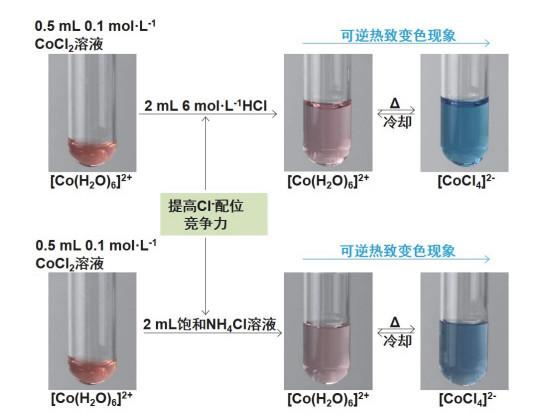## Thermochromic Phenomena in "Elemental Chemistry Experiment" (Ⅰ): Thermochromic Phenomenon and Discussion on Thermochromic Mechanism of Some Co(Ⅱ) Compounds

Ruan Chanzi, Pan Rui, Xu Zhenling, Weng Yuhua, Zhang Chunyan, Lü Yinyun, Dong Zhiqiang, Ren Yanping,

 基金资助: 2021年度教育部“基础学科拔尖学生培养计划2.0”研究课题.  20212058教育部2020年产学合作协同育人项目.  202002060029首批国家级线下一流课程建设项目Abstract

In the Co2+ identification experiment, it was accidentally found that with an appropriate amount of SCN- added into CoCl2 solution, the solution presented reversible thermochromic phenomenon. Inspired by this phenomenon, the "flipped classroom" mode of experimental teaching of "doing first, teaching later, teaching determined by doing" was adopted accordingly based on the analysis and discussion of its thermochromic mechanism. Specifically, students do the experiment first and have questions during the experimental process, then the teacher demonstrates with students based on their questions, leading students to intuitive understanding of the thermochromic phenomenon and its influencing factors of some Co(Ⅱ) compounds or complexes. This article inspires students to use chemical principles and knowledge to explain and discuss the nature of thermochromic phenomenon, so as to deepen students' understanding of the structure, properties and concept of splitting energy of complexes. It also cultivates the students' ability of observation, analysis, judgment, induction, reasoning, summary, conclusion regular and "critical" thinking.

Keywords： Co(Ⅱ) compounds ; Thermochromism ; Influencing factors ; Discussion on thermochromic mechanism

Ruan Chanzi. Thermochromic Phenomena in "Elemental Chemistry Experiment" (Ⅰ): Thermochromic Phenomenon and Discussion on Thermochromic Mechanism of Some Co(Ⅱ) Compounds. University Chemistry[J], 2022, 37(1): 2104004-0 doi:10.3866/PKU.DXHX202104004

## 1 CoCl2与NH4SCN混合溶液体系的热致变色现象

### 图1$\underset{粉红色}{\mathop{{{\left[ \text{Co}{{\left( {{\text{H}}_{2}}\text{O} \right)}_{6}} \right]}^{2+}}}}\, +\text{SC}{{\text{N}}^{-}}\rightleftarrows \underset{紫红色\left( n=1-3 \right)}{\mathop{{{\left[ \text{Co}{{(\text{SCN})}_{n}}{{\left( {{\text{H}}_{2}}\text{O} \right)}_{4-n}} \right]}^{2-n}}}}\, \underset{冷却}{\overset{\Delta }{\mathop{\rightleftarrows }}}\, \underset{蓝色}{\mathop{{{\left[ \text{Co}{{(\text{SCN})}_{4}} \right]}^{2-}}}}\,$

### 图2### 2.1 0.1 mol·L−1 CoCl2溶液的热致变色现象

0.1 mol·L−1 CoCl2溶液是否具有热致变色现象？消除学生质疑的最好方法不是争辩，还是通过图 2所示的演示实验事实来说明。

### 图3$\underset{红色 }{\mathop{{{\left[ \text{Co}{{\left( {{\text{H}}_{2}}\text{O} \right)}_{6}} \right]}^{2+}}}}\, +4\text{C}{{\text{l}}^{-}}\stackrel{\Delta}{\underset{\text { 冷却 }}{\rightleftarrows}} \underset{蓝色}{\mathop{{{\left[ \text{CoC}{{\text{l}}_{4}} \right]}^{2-}}}}\, +6{{\text{H}}_{2}}\text{O}$

### 图4### 图5#### 2.2.3 C2H5OH对CoCl2溶液热致变色现象的影响

$\underset{红色}{\mathop{{{\left[ \text{Co}{{\left( {{\text{H}}_{2}}\text{O} \right)}_{6}} \right]}^{2+}}}}\, +4\text{C}{{\text{l}}^{-}}+6{{\text{C}}_{2}}{{\text{H}}_{5}}\text{OH}\underset{\text{ 冷却 }}{\mathop{\overset{\Delta }{\mathop{\rightleftarrows }}\, }}\, \underset{\text{ }}{\mathop{\underset{\text{蓝色}}{\mathop{{{\left[ \text{CoC}{{\text{l}}_{4}} \right]}^{2-}}}}\, +6{{\text{C}}_{2}}{{\text{H}}_{5}}\text{OH}-{{\text{H}}_{2}}\text{O}}}\,$

### 图6$\underset{红色}{\mathop{{{\left[ \text{Co}{{\left( {{\text{H}}_{2}}\text{O} \right)}_{6}} \right]}^{2+}}}}\, +4\text{C}{{\text{l}}^{-}}+6{{\text{C}}_{2}}{{\text{H}}_{5}}\text{OH}\underset{\text{ 冷却 }}{\mathop{\overset{\Delta }{\mathop{\rightleftarrows }}\, }}\, \underset{蓝色}{\mathop{\text{Co}{{\left( {{\text{C}}_{2}}{{\text{H}}_{5}}\text{OH} \right)}_{2}}\text{C}{{\text{l}}_{2}}}}\, +6{{\text{H}}_{2}}\text{O}$

## 3 Co(NO3)2和CoSO4溶液的热致变色现象

### 图7### 图8Cr3+有3个成单的d电子，吸收部分可见光可以发生dd跃迁，所以Cr(Ⅲ)化合物都显有颜色。那么，常见Cr(Ⅲ)化合物是否具有热致变色现象？其热致变色的机理又是什么？我们将继续以演示实验为基础，观察和记录常见Cr(Ⅲ)化合物的热致变色现象，分析和探讨其热致变色机理，希望进一步给学生有益的借鉴和启发。

## 参考文献 原文顺序 文献年度倒序 文中引用次数倒序 被引期刊影响因子

/

 〈〉